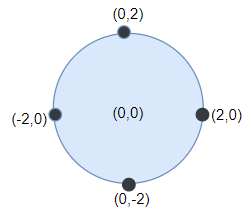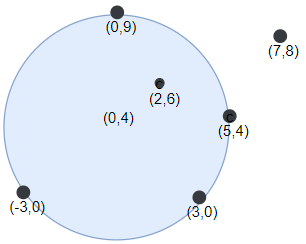# GeetCode Hub

You have a very large square wall and a circular dartboard placed on the wall. You have been challenged to throw darts into the board blindfolded. Darts thrown at the wall are represented as an array of `points` on a 2D plane.

Return the maximum number of points that are within or lie on any circular dartboard of radius `r`.

Example 1:```Input: points = [[-2,0],[2,0],[0,2],[0,-2]], r = 2
Output: 4
Explanation: Circle dartboard with center in (0,0) and radius = 2 contain all points.
```

Example 2:```Input: points = [[-3,0],[3,0],[2,6],[5,4],[0,9],[7,8]], r = 5
Output: 5
Explanation: Circle dartboard with center in (0,4) and radius = 5 contain all points except the point (7,8).
```

Example 3:

```Input: points = [[-2,0],[2,0],[0,2],[0,-2]], r = 1
Output: 1
```

Example 4:

```Input: points = [[1,2],[3,5],[1,-1],[2,3],[4,1],[1,3]], r = 2
Output: 4
```

Constraints:

• `1 <= points.length <= 100`
• `points[i].length == 2`
• `-10^4 <= points[i], points[i] <= 10^4`
• `1 <= r <= 5000`

class Solution { public int numPoints(int[][] points, int r) { } }